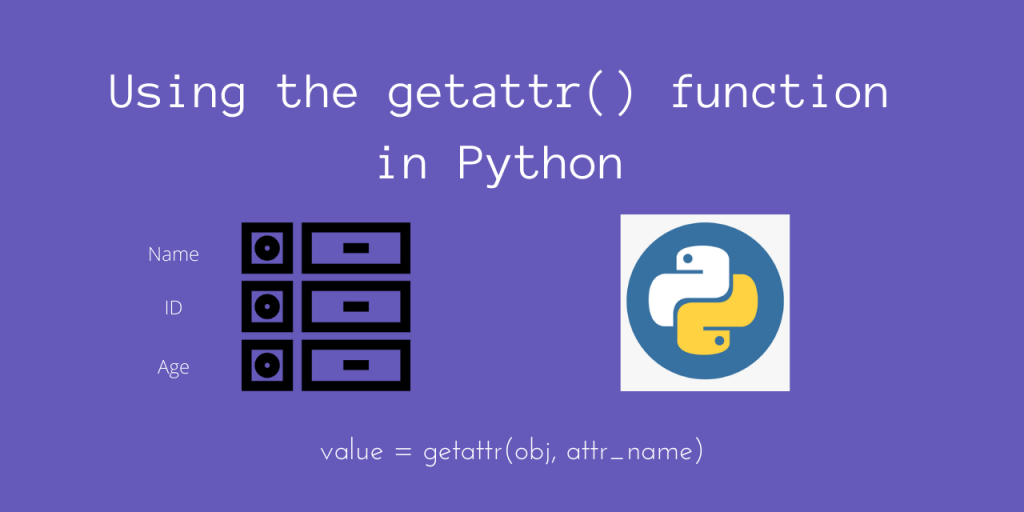# Using the Python getattr() functionHello again! In today’s article, we’ll be looking at the Python getattr() function in Python.

This function tries to get the attribute of a Python object. This is very similar to the setattr() function, but it does not modify the object.

Let’s find out how we can put this function to use, using some simple examples.

## Basic Syntax of Python getattr()

The basic syntax of the getattr() function is as follows:

```value = getattr(object, attribute_name[, default_value])
```

Here, we pass `object` to `getattr()`. It tries to fetch `attribute_name` (which must be a string) of this object. If the attribute exists, it will give us the corresponding `value`.

This is equivalent to the syntax:

```value = object.attribute_name
```

Otherwise, there are two cases:

• If the default value (`default_value`) is provided, it will simply use it and return the value of this default value.
• Otherwise, it will simply raise an `AttributeError` Exception, since our attribute is not found.

The point of `getattr()` is to not only get the attribute value, but to also check that it exists!

Let’s now look at a few examples, to better our understanding.

## Using getattr() – Some Examples

Let’s take a simple example first, where you have a Student class, with `name` and `roll_num` attributes. We’ll get them using `getattr()`.

```class Student():
def __init__(self, name, roll_no):
self.name = name
self.roll_no = roll_no

student = Student('Amit', 8)

print(f"Name: {getattr(student, 'name')}")
print(f"Roll No: {getattr(student, 'roll_no')}")
```

Output

```Name: Amit
Roll No: 8
```

Indeed, we did set the correct attributes, and we get them back using `getattr()`!

Now, in this case, since both the attributes were there, no errors occurred. However, let’s try to get an attribute which we haven’t put inside the class; for example – `age`.

```class Student():
def __init__(self, name, roll_no):
self.name = name
self.roll_no = roll_no

student = Student('Amit', 8)

print(f"Name: {getattr(student, 'name')}")
print(f"Roll No: {getattr(student, 'roll_no')}")

# Will raise 'AttributeError' Exception since the attribute 'age' is not defined for our instance
print(f"Age: {getattr(student, 'age')}")
```

Output

```Name: Amit
Roll No: 8
Traceback (most recent call last):
File "getattr_example.py", line 12, in <module>
print(f"Age: {getattr(student, 'age')}")
AttributeError: 'Student' object has no attribute 'age'
```

Here, we tried to retrieve an attribute which wasn’t defined. Since there were no default options, Python directly raised an Exception.

If we want to set the default option to `'100'`, then in this case, although we try to get `age`, since it isn’t there, it will return `100 `instead.

Let’s test it out.

```class Student():
def __init__(self, name, roll_no):
self.name = name
self.roll_no = roll_no

student = Student('Amit', 8)

print(f"Name: {getattr(student, 'name')}")
print(f"Roll No: {getattr(student, 'roll_no')}")

# Will not raise AttributeError, since a default value is provided
print(f"Age: {getattr(student, 'age', 100)}")
```

Output

```Name: Amit
Roll No: 8
Age: 100
```

## Why should you use getattr() ?

Since we mentioned earlier that this function is equivalent to `object.attribute_name`, what is the point of this function?

While the above statement using dot notation is true, it is valid only when the attribute name is actually defined at the time of calling!

So, if your classes are such that the object structure keeps changing from time to time, you could use `getattr()` to check whether the object is in some particular state!

It also gives us a very good way to easily handle exceptions and also provide fallback default values. In case something went wrong, we could either catch the `AttributeError` exception or inspect the default value.

Hope that makes it a bit more clearer as to why this may be useful for you!

## Conclusion

We learned about how we could use the `getattr()` function to retrieve the attributes of an object during runtime.